HashMap

# 散列表中维护了一个数组，数组的每一个元素被称为一个桶（bucket），当你传入一个key

"a"进行查询时，散列表会先把key传入散列（hash）函数中进行寻址，得到的结果就是数组的下标，然后再通过这个下标访问数组即可得到相关联的值。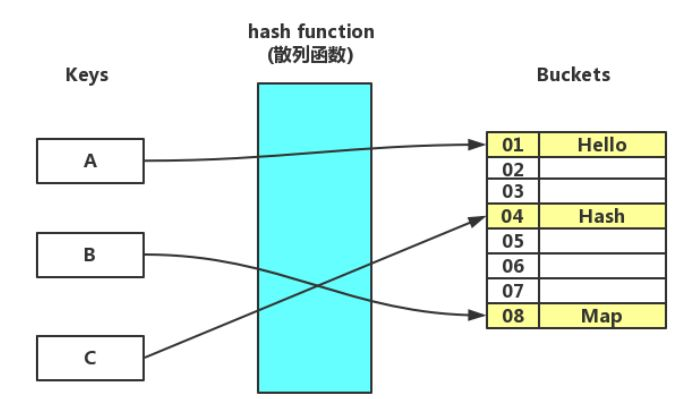hash算法是一种可以从任何数据中提取出其“指纹”的数据摘要算法，它将任意大小的数据（输入）映射到一个固定大小的序列（输出）上，这个序列被称为hash code、数据摘要或者指纹。比较出名的hash算法有MD5、SHA。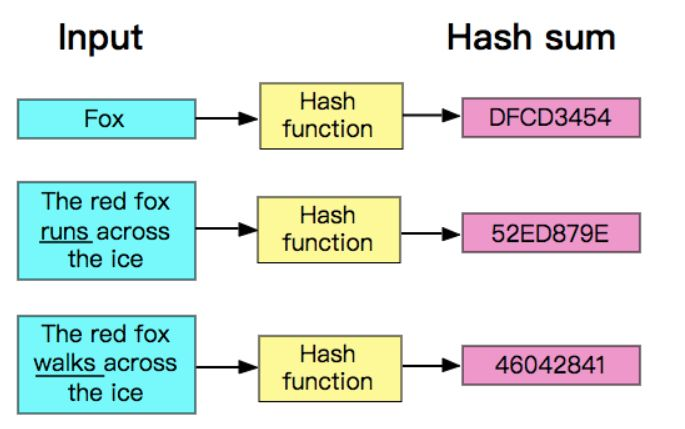hash是具有唯一性且不可逆的，唯一性指的是相同的输入产生的hash code永远是一样的，而不可逆也比较容易理解，数据摘要算法并不是压缩算法，它只是生成了一个该数据的摘要，没有将数据进行压缩。压缩算法一般都是使用一种更节省空间的编码规则将数据重新编码，解压缩只需要按着编码规则解码就是了，试想一下，一个几百MB甚至几GB的数据生成的hash code都只是一个拥有固定长度的序列，如果再能逆向解压缩，那么其他压缩算法该情何以堪？

private int hash(Key key) {

// & 0x7fffffff 是为了屏蔽符号位，M为bucket数组的长度

return (key.hashCode() & 0x7fffffff) % M;

}

/** The value is used for character storage. */

private final char value[];

/** Cache the hash code for the string */

private int hash; // Default to 0

public int hashCode() {

int h = hash;

if (h == 0 && value.length > 0) {

char val[] = value;

for (int i = 0; i < value.length; i++) {

h = 31 * h + val[i];

}

hash = h;

}

return h;

}

HashMap没有采用这么简单的方法，有一个原因是HashMap中的buckets数组的长度永远为一个2的幂，而不是一个素数，如果长度为素数，那么可能会更适合简单暴力的除留余数法（当然除留余数法虽然简单却并不是那么高效的），顺便一提，时代的眼泪Hashtable就使用了除留余数法，它没有强制约束buckets数组的长度。

HashMap在内部实现了一个hash()函数，首先要对hashCode()的返回值进行处理：

static final int hash(Object key) {

int h;

return (key == null) ? 0 : (h = key.hashCode()) ^ (h >>> 16);

}

• 1做与运算（table为buckets数组），得到的结果即是数组的下标。

table.length -

## 1就像是一个低位掩码（这个设计也优化了扩容操作的性能），它和hash()做与操作时必然会将高位屏蔽（因为一个HashMap不可能有特别大的buckets数组，至少在不断自动扩容之前是不可能的，所以table.length

1的大部分高位都为0），只保留低位，看似没什么毛病，但这其实暗藏玄机，它会导致总是只有最低的几位是有效的，这样就算你的hashCode()实现得再好也难以避免发生碰撞。这时，hash()函数的价值就体现出来了，它对hash code的低位添加了随机性并且混合了高位的部分特征，显著减少了碰撞冲突的发生（关于hash()函数的效果如何，可以参考这篇文章An introduction to optimising a hashing strategy）。

HashMap的散列函数具体流程如下图：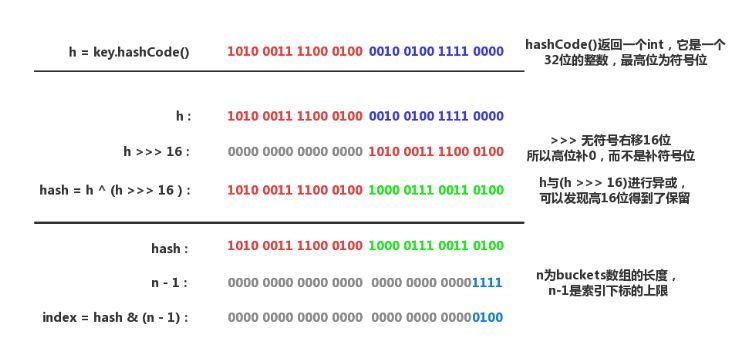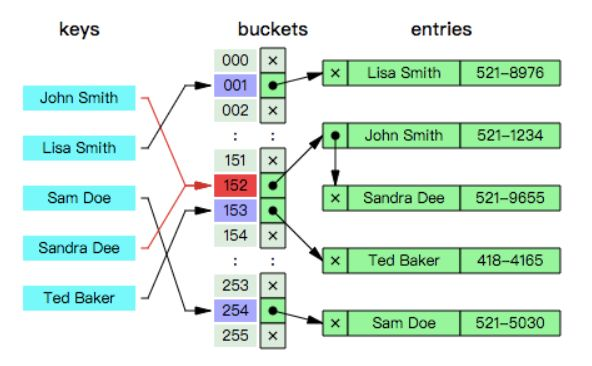/**

* The bin count threshold for using a tree rather than list for a

* bin. Bins are converted to trees when adding an element to a

* bin with at least this many nodes. The value must be greater

* than 2 and should be at least 8 to mesh with assumptions in

* tree removal about conversion back to plain bins upon

* shrinkage.

*/

static final int TREEIFY_THRESHOLD = 8;

/**

* 替换指定hash所处位置的链表中的所有节点为TreeNode，

* 如果buckets数组太小，就进行扩容。

*/

final void treeifyBin(Node<K,V>[] tab, int hash) {

int n, index; Node<K,V> e;

// MIN_TREEIFY_CAPACITY = 64，小于该值代表数组中的节点并不是很多

// 所以选择进行扩容，只有数组长度大于该值时才会进行树化。

if (tab == null || (n = tab.length) < MIN_TREEIFY_CAPACITY)

resize();

else if ((e = tab[index = (n - 1) & hash]) != null) {

TreeNode<K,V> hd = null, tl = null;

// 转换链表节点为树节点，注意要处理好连接关系

do {

TreeNode<K,V> p = replacementTreeNode(e, null);

if (tl == null)

hd = p;

else {

p.prev = tl;

tl.next = p;

}

tl = p;

} while ((e = e.next) != null);

if ((tab[index] = hd) != null)

hd.treeify(tab); // 从头部开始构造树

}

}

// 该函数定义在TreeNode中

final void treeify(Node<K,V>[] tab) {

TreeNode<K,V> root = null;

for (TreeNode<K,V> x = this, next; x != null; x = next) {

next = (TreeNode<K,V>)x.next;

x.left = x.right = null;

if (root == null) { // 初始化root节点

x.parent = null;

x.red = false;

root = x;

}

else {

K k = x.key;

int h = x.hash;

Class<?> kc = null;

for (TreeNode<K,V> p = root;;) {

int dir, ph;

K pk = p.key;

// 确定节点的方向

if ((ph = p.hash) > h)

dir = -1;

else if (ph < h)

dir = 1;

// 如果kc == null

// 并且k没有实现Comparable接口

// 或者k与pk是没有可比较性的（类型不同）

// 或者k与pk是相等的（返回0也有可能是相等）

else if ((kc == null &&

(kc = comparableClassFor(k)) == null) ||

(dir = compareComparables(kc, k, pk)) == 0)

dir = tieBreakOrder(k, pk);

// 确定方向后插入节点，修正红黑树的平衡

TreeNode<K,V> xp = p;

if ((p = (dir <= 0) ? p.left : p.right) == null) {

x.parent = xp;

if (dir <= 0)

xp.left = x;

else

xp.right = x;

root = balanceInsertion(root, x);

break;

}

}

}

}

// 确保给定的root是该bucket中的第一个节点

moveRootToFront(tab, root);

}

static int tieBreakOrder(Object a, Object b) {

int d;

if (a == null || b == null ||

(d = a.getClass().getName().

compareTo(b.getClass().getName())) == 0)

// System.identityHashCode()将调用并返回传入对象的默认hashCode()

// 也就是说，无论是否重写了hashCode()，都将调用Object.hashCode()。

// 如果传入的对象是null，那么就返回0

d = (System.identityHashCode(a) <= System.identityHashCode(b) ?

-1 : 1);

return d;

}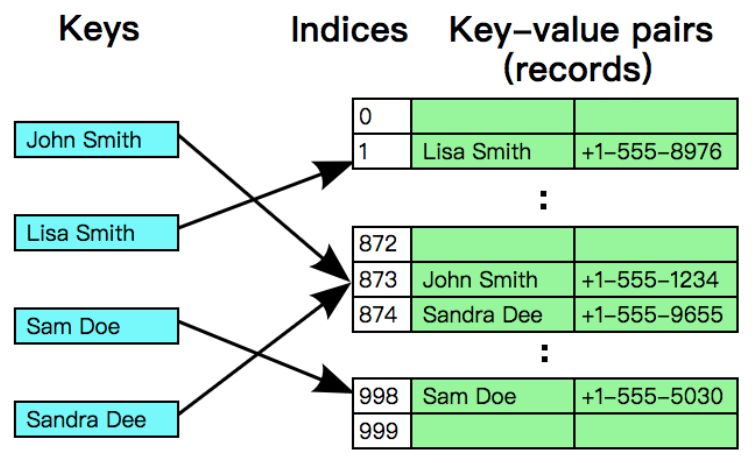private K[] keys; // 存储key的数组

private V[] vals; // 存储值的数组

public V get(K key) {

// m是buckets数组的长度，即keys和vals的长度。

// 当i等于m时，取模运算会得0（折回数组头部）

for (int i = hash(key); keys[i] != null; i = (i + 1) % m) {

if (keys[i].equals(key))

return vals[i];

}

return null;

}

public void put(K key, V value) {

// n是Entry的数量，如果n超过了数组长度的一半，就扩容一倍

if (n >= m / 2) resize(2 * m);

int i;

for (i = hash(key); keys[i] != null; i = (i + 1) % m) {

if (keys[i].equals(key)) {

vals[i] = value;

return;

}

}

// 没有找到目标，那么就插入一对新的Entry

keys[i] = key;

vals[i] = value;

n++;

}

public V delete(K key) {

int i = hash(key);

// 先找到目标的索引

while (!key.equals(keys[i])) {

i = (i + 1) % m;

}

V oldValue = vals[i];

// 删除目标key和value

keys[i] = null;

vals[i] = null;

// 指针移动到下一个索引

i = (i + 1) % m;

while (keys[i] != null) {

// 先删除然后重新插入

K keyToRehash = keys[i];

V valToRehash = vals[i];

keys[i] = null;

vals[i] = null;

n--;

put(keyToRehash, valToRehash);

i = (i + 1) % m;

}

n--;

// 当前Entry小于等于数组长度的八分之一时，进行缩容

if (n > 0 && n <= m / 8) resize(m / 2);

return oldValue;

}

/**

* 可以发现构造函数中根本就没初始化buckets数组。

* （之前说过buckets数组会推迟到第一次调用put()时进行初始化）

*/

public HashMap(int initialCapacity, float loadFactor) {

if (initialCapacity < 0)

throw new IllegalArgumentException("Illegal initial capacity: " +

initialCapacity);

if (initialCapacity > MAXIMUM_CAPACITY)

initialCapacity = MAXIMUM_CAPACITY;

throw new IllegalArgumentException("Illegal load factor: " +

// tableSizeFor()确保initialCapacity必须为一个2的N次方

this.threshold = tableSizeFor(initialCapacity);

}

buckets数组的大小约束对于整个HashMap都至关重要，为了防止传入一个不是2次幂的整数，必须要有所防范。tableSizeFor()函数会尝试修正一个整数，并转换为离该整数最近的2次幂。

/**

* Returns a power of two size for the given target capacity.

*/

static final int tableSizeFor(int cap) {

int n = cap - 1;

n |= n >>> 1;

n |= n >>> 2;

n |= n >>> 4;

n |= n >>> 8;

n |= n >>> 16;

return (n < 0) ? 1 : (n >= MAXIMUM_CAPACITY) ? MAXIMUM_CAPACITY : n + 1;

}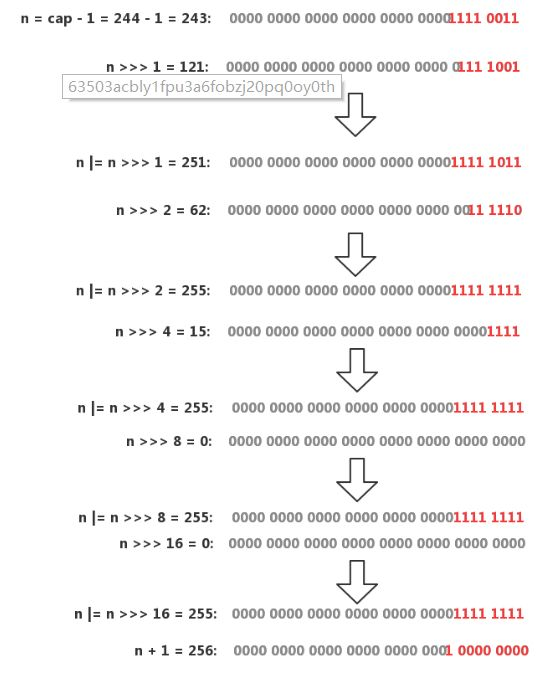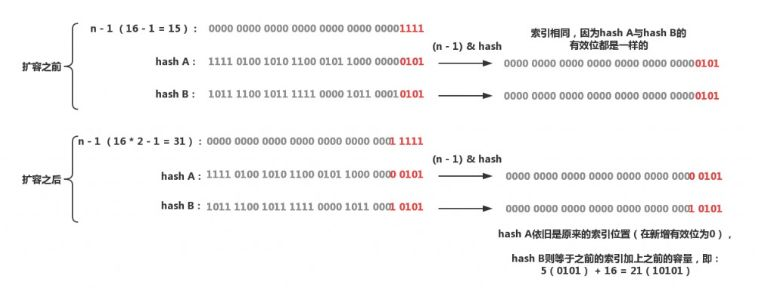final Node<K,V>[] resize() {

Node<K,V>[] oldTab = table; // table就是buckets数组

int oldCap = (oldTab == null) ? 0 : oldTab.length;

int oldThr = threshold;

int newCap, newThr = 0;

// oldCap大于0，进行扩容，设置阈值与新的容量

if (oldCap > 0) {

// 超过最大值不会进行扩容，并且把阈值设置成Interger.MAX_VALUE

if (oldCap >= MAXIMUM_CAPACITY) {

threshold = Integer.MAX_VALUE;

return oldTab;

}

// 没超过最大值，扩容为原来的2倍

// 向左移1位等价于乘2

else if ((newCap = oldCap << 1) < MAXIMUM_CAPACITY &&

oldCap >= DEFAULT_INITIAL_CAPACITY)

newThr = oldThr << 1; // double threshold

}

// oldCap = 0，oldThr大于0，那么就把阈值做为新容量以进行初始化

// 这种情况发生在用户调用了带有参数的构造函数（会对threshold进行初始化）

else if (oldThr > 0) // initial capacity was placed in threshold

newCap = oldThr;

// oldCap与oldThr都为0，这种情况发生在用户调用了无参构造函数

// 采用默认值进行初始化

else { // zero initial threshold signifies using defaults

newCap = DEFAULT_INITIAL_CAPACITY;

}

// 如果newThr还没有被赋值，那么就根据newCap计算出阈值

if (newThr == 0) {

float ft = (float)newCap * loadFactor;

newThr = (newCap < MAXIMUM_CAPACITY && ft < (float)MAXIMUM_CAPACITY ?

(int)ft : Integer.MAX_VALUE);

}

threshold = newThr;

style="font-size: 16px;">@SuppressWarnings({"rawtypes","unchecked"})

Node<K,V>[] newTab = (Node<K,V>[])new Node[newCap];

table = newTab;

// 如果oldTab != null，代表这是扩容操作

// 需要将扩容前的数组数据迁移到新数组

if (oldTab != null) {

// 遍历oldTab的每一个bucket，然后移动到newTab

for (int j = 0; j < oldCap; ++j) {

Node<K,V> e;

if ((e = oldTab[j]) != null) {

oldTab[j] = null;

// 索引j的bucket只有一个Entry（未发生过碰撞）

// 直接移动到newTab

if (e.next == null)

newTab[e.hash & (newCap - 1)] = e;

// 如果是一个树节点（代表已经转换成红黑树了）

// 那么就将这个节点拆分为lower和upper两棵树

// 首先会对这个节点进行遍历

// 只要当前节点的hash & oldCap == 0就链接到lower树

// 注意这里是与oldCap进行与运算，而不是oldCap - 1(n - 1)

// oldCap就是扩容后新增有效位的掩码

// 比如oldCap=16，二进制10000，n-1 = 1111，扩容后的n-1 = 11111

// 只要hash & oldCap == 0，就代表hash的新增有效位为0

// 否则就链接到upper树（新增有效位为1）

// lower会被放入newTab[原索引j]，upper树会被放到newTab[原索引j + oldCap]

// 如果lower或者upper树的节点少于阈值，会被退化成链表

else if (e instanceof TreeNode)

((TreeNode<K,V>)e).split(this, newTab, j, oldCap);

else { // preserve order

// 下面操作的逻辑与分裂树节点基本一致

// 只不过split()操作的是TreeNode

// 而且会将两条TreeNode链表组织成红黑树

Node<K,V> loHead = null, loTail = null;

Node<K,V> hiHead = null, hiTail = null;

Node<K,V> next;

do {

next = e.next;

if ((e.hash & oldCap) == 0) {

if (loTail == null)

else

loTail.next = e;

loTail = e;

}

else {

if (hiTail == null)

else

hiTail.next = e;

hiTail = e;

}

} while ((e = next) != null);

if (loTail != null) {

loTail.next = null;

}

if (hiTail != null) {

hiTail.next = null;

}

}

}

}

}

return newTab;

}public V put(K key, V value) {

return putVal(hash(key), key, value, false, true);

}

final V putVal(int hash, K key, V value, boolean onlyIfAbsent,

boolean evict) {

Node<K,V>[] tab; Node<K,V> p; int n, i;

// table == null or table.length == 0

// 第一次调用put()，初始化table

if ((tab = table) == null || (n = tab.length) == 0)

n = (tab = resize()).length;

// 没有发生碰撞，直接放入到数组

if ((p = tab[i = (n - 1) & hash]) == null)

tab[i] = newNode(hash, key, value, null);

else {

Node<K,V> e; K k;

// 发生碰撞（头节点就是目标节点）

if (p.hash == hash &&

((k = p.key) == key || (key != null && key.equals(k))))

e = p;

// 节点为红黑树

else if (p instanceof TreeNode)

e = ((TreeNode<K,V>)p).putTreeVal(this, tab, hash, key, value);

// 节点为链表

else {

for (int binCount = 0; ; ++binCount) {

// 未找到目标节点，在链表尾部链接新节点

if ((e = p.next) == null) {

p.next = newNode(hash, key, value, null);

if (binCount >= TREEIFY_THRESHOLD - 1) // -1 for 1st

// 链表过长，转换为红黑树

treeifyBin(tab, hash);

break;

}

// 找到目标节点，退出循环

if (e.hash == hash &&

((k = e.key) == key || (key != null && key.equals(k))))

break;

p = e;

}

}

// 节点已存在，替换value

if (e != null) { // existing mapping for key

V oldValue = e.value;

if (!onlyIfAbsent || oldValue == null)

e.value = value;

// 在HashMap中没有意义

afterNodeAccess(e);

return oldValue;

}

}

++modCount;

// 超过临界值，进行扩容

if (++size > threshold)

resize();

afterNodeInsertion(evict);

return null;

}

【关于投稿】